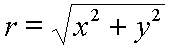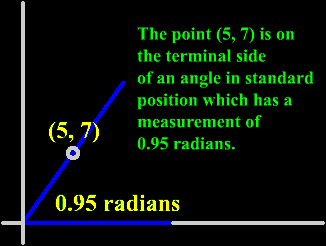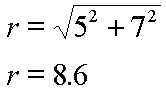﻿ Trigonometry Function Point Definitions | Zona Land Education

# Trig Function Point Definitions

This program shows the relationship between two graphs. The left graph is the common (x, y) graph. A point on this graph is used to calculate the value for a trigonometry function. The other graph shows the value for the trigonometry function versus the input angle. The angle under consideration is the same for both graphs. The trig function is selected with the radio buttons above the graphs. Calculations for the values are also shown.

More Quick Instructions and Further Discussion below...

a = ? x = ? y = ? r = ?
a = ? sin(a) = ?
sin(a) = y / r
sin(??.??) = ??.?? / ??.??
sin(??.??) = ??.??

Quick Instructions:

• The above program starts with the sine function active. Any of the six trigonometric functions can be activated by choosing the appropriate radio button at the top of the program.
• The large square graph on the left is the (x, y) coordinate plane. It extends from -10.0 to +10.0 along the x-axis and the y-axis. This graph's grid is marked every unit distance.
• The upper right rectangular graph shows the graph of the currently active trig function. For example, when the sine function is active, this graph shows the sin(a) vs. a, where a represents the angle. Horizontally, this graph has a domain from 0 radians to 2pi radians (0 to 360 degrees) with markers every pi/2 radians (90 degrees). Vertically, the range of this graph runs from -2.0 to +2.0 for the sine and cosine functions, and it runs from -5.0 to +5.0 for the tangent, cotangent, secant and cosecant functions. Vertical marking occur every unit distance.
• The lower right area shows the calculation for the trig function at the angle defined by the (x, y) coordinates.
• With the sine function radio button is selected, click (or tap) somewhere on the left (x, y) graph. Notice:
• The (x, y) coordinates and the radius (r) are presented. X is in red, y is in green, r is in blue.
• The angle whose terminal side goes through this (x, y) point is drawn. This angle is colored magenta. Its value is presented in the upper left corner of the (x, y) graph.
• The relevant quantities necessary for the sine calculation are highlighted on the graph, (the y distance and the radius in this case). The values for these quantities are presented near their graphic representations.
• The upper right hand graph shows the current function - the sine function. The current input angle and the current output value for this function are presented.
• In the lower right the value for the trig function at the current angle is calculated using the relevant quantities from the (x, y) graph.
• All values are rounded to two decimal places. Certainly more precise values for the trig functions are available elsewhere. This program, though, is not meant to be a calculator. It is meant to demonstrate the relationships among several trigonometric concepts.
• Try different (x, y) positions and different trig functions.

Further Discussion:

This material explains the definitions of the six trigonometric functions in terms of an (x, y) point located on the terminal side of the input angle. You should be familiar with:

The six trigonometric functions (sine, cosine, tangent, cotangent, secant and cosecant) are usually thought to accept an angle as input and output a pure number. For the purposes of the definitions this angle is to be placed in standard position. We will be concerned with any (x, y) point located on the terminal side of this angle. These definitions are based on such an (x, y) point.

These definitions also use the distance from the origin to the (x, y) point. This distance will be referred to as r (for radius) and can be calculated like this:The six definitions are:

sin(angle) = y/r

cos(angle) = x/r

tan(angle) = y/x (x not equal to zero)

csc(angle) = r/y (y not equal to zero)

sec(angle) = r/x (x not equal to zero)

cot(angle) = x/y (y not equal to zero)

So, for example, the point (5, 7) is on the terminal side of an angle in standard position which has a measure of about 0.95 radians (about 54 degrees):The distance from the origin to the point (5, 7) can be calculated this way (approximate result given):Therefore, the six trigonometric function values for this angle can be calculated as follows (approximate results given):

sin(0.95) = y/r = 7/8.6 = 0.81

cos(0.95) = x/r = 5/8.6 = 0.58

tan(0.95) = y/x = 7/5 = 1.4

csc(0.95) = r/y = 8.6/7 = 1.2

sec(0.95) = r/x = 8.6/5 = 1.7

cot(0.95) = x/y = 5/7 = 0.71

In all of the above calculations approximate results were given. Of course, if you are doing more careful and important work you would use measurements and calculations of higher significance.

The definitions given here for the six trigonometric functions are more powerful than the right triangle definitions given in the introduction to trigonometry section. The right triangle definitions are only good for angles up to pi/2 radians (90 degrees). These trig definitions based upon an (x, y) point on the terminal side of an angle are good for angles of any measurement, positive or negative.

Custom Search